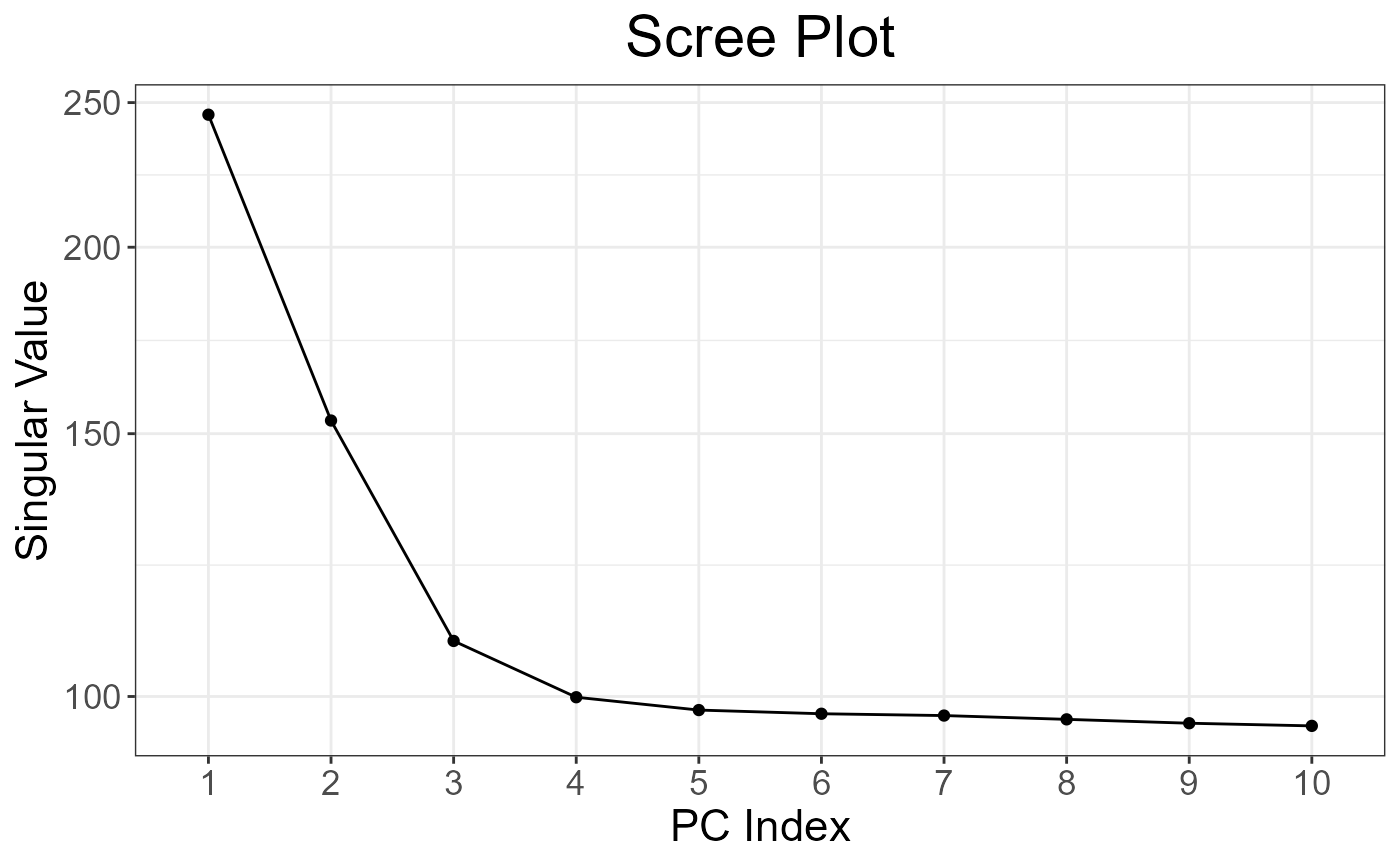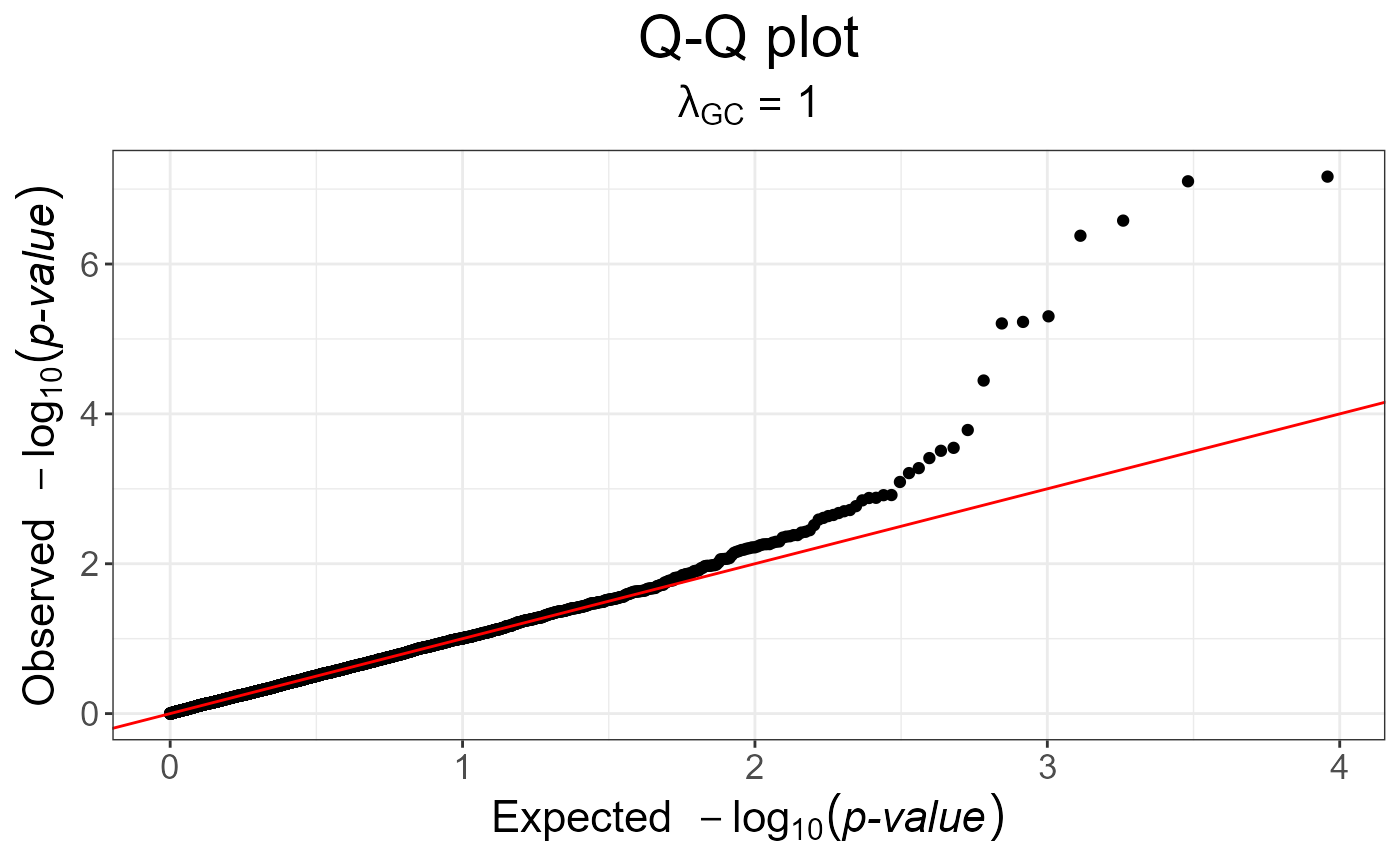Method to detect genetic markers involved in biological adaptation. This provides a statistical tool for outlier detection based on Principal Component Analysis. This corresponds to the statistic based on mahalanobis distance, as implemented in package pcadapt.

snp_pcadapt(
G,
U.row,
ind.row = rows_along(G),
ind.col = cols_along(G),
ncores = 1
)

obj.bed,
U.row,
ind.row = rows_along(obj.bed),
ind.col = cols_along(obj.bed),
ncores = 1
)

G

A FBM.code256 (typically <bigSNP>$genotypes). You shouldn't have missing values. Also, remember to do quality control, e.g. some algorithms in this package won't work if you use SNPs with 0 MAF. U.row Left singular vectors (not scores, $$U^T U = I$$) corresponding to ind.row. ind.row An optional vector of the row indices (individuals) that are used. If not specified, all rows are used. Don't use negative indices. ind.col An optional vector of the column indices (SNPs) that are used. If not specified, all columns are used. Don't use negative indices. ncores Number of cores used. Default doesn't use parallelism. You may use nb_cores. obj.bed Object of type bed, which is the mapping of some bed file. Use obj.bed <- bed(bedfile) to get this object. ## Value An object of classes mhtest and data.frame returning one score by SNP. See methods(class = "mhtest"). ## See also ## Examples test <- snp_attachExtdata() G <- test$genotypes
obj.svd <- big_SVD(G, fun.scaling = snp_scaleBinom(), k = 10)
plot(obj.svd) # there seems to be 3 "significant" components